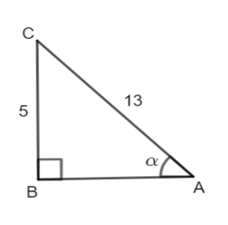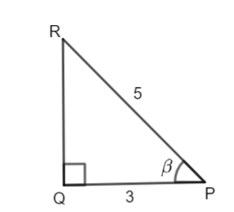#### Please solve RD Sharma Chapter Inverse trigonometric functions exercise 3.8 question 2 sub question (iii) maths text book solutionHint:

We convert expression in the form of  so we know the formula of it.

Given:

We have to prove

Solution:

LHS =

…(i)

But we have not  and  . So, we find out first then we put in equation (i)

Let, in ,  angle and right angle at B.[we will ignore the -ve sign because AB is a length and it can’t be -ve]

Let, in ,  angle  and right angle at B.Now,                                                         [ put,  and ]

[ we take LCM of 3 and 12]

Hence proved.

#### infoexpert24